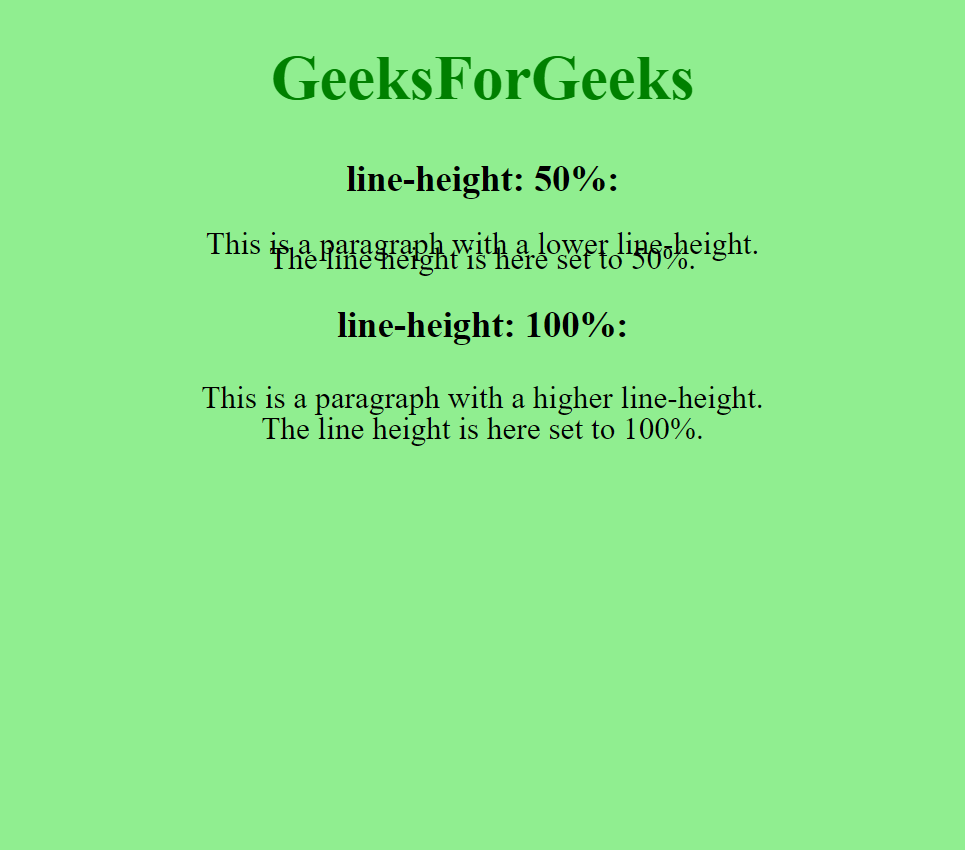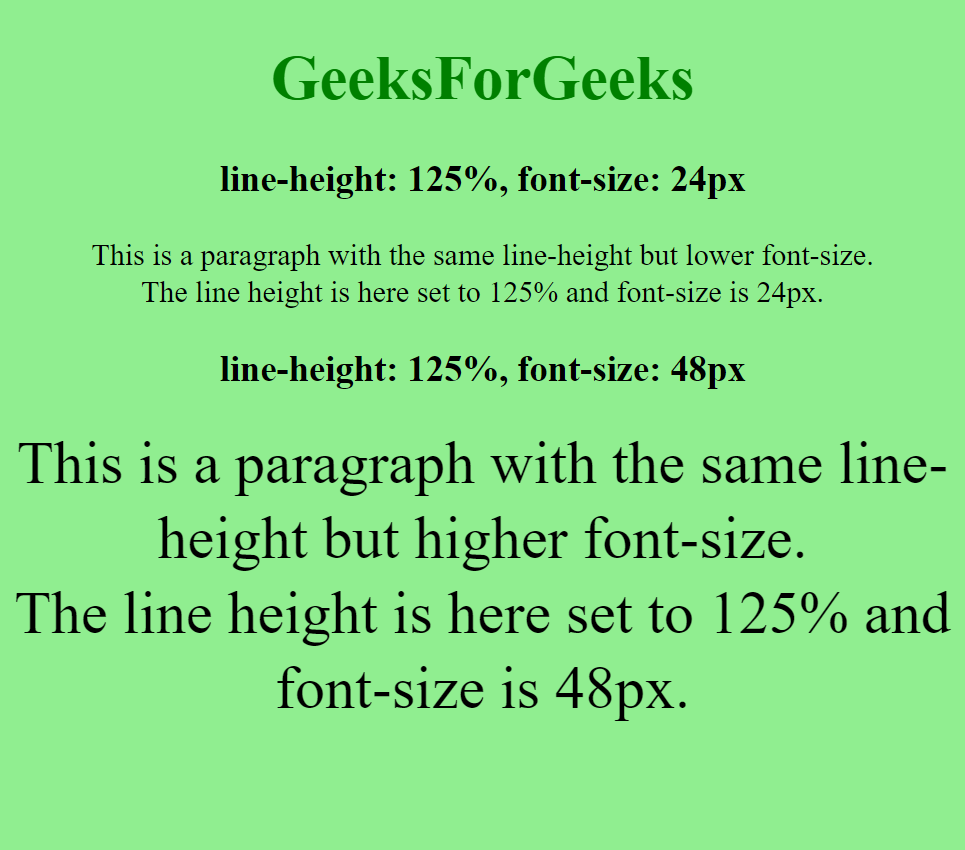# How to set line height in percent using CSS ?

• Last Updated : 18 Apr, 2021

In this article, we will learn how to set the line height in percent using CSS. Line height is a property of CSS that is used to provide height after every element.

Approach: We will use the line-height property in CSS and set the value using a percentage. The percentage sets the line height in relation to the font size of the element. The final value that is set is determined is by multiplying the element’s computed font size with the given percentage value.

Syntax:

`line-height: percent`

Example 1: In this example, different percentage values of the line-height are used with the font-size being the same.

## HTML

 ```<``html``>`` ` `<``head``>``    ``<``style``>``        ``body {``            ``text-align: center;``            ``font-size: 25px;``            ``background-color: lightgreen;``        ``}`` ` `        ``div.a {``            ``line-height: 50%;``        ``}`` ` `        ``div.b {``            ``line-height: 100%;``        ``}``    `````` ` `<``body``>`` ` `    ``<``h1` `style``=``"color:green"``>``        ``GeeksForGeeks``    ```` ` `    ``<``h3``>line-height: 50%:``    ``<``div` `class``=``"a"``>``        ``This is a paragraph with a``        ``lower line-height.<``br``>``        ``The line height is here set to 50%.``    ```` ` `    ``<``h3``>line-height: 100%:``    ``<``div` `class``=``"b"``>``        ``This is a paragraph with a``        ``higher line-height.<``br``>The ``        ``line height is here set to 100%.``    `````` ` ``

Output:Example 2: In this example, the same percentage value of the line-height is used with different font-size.

## HTML

 ```<``html``>`` ` `<``head``>``    ``<``style``>``        ``body {``            ``text-align: center;``            ``font-size: 25px;``            ``background-color: lightgreen;``        ``}`` ` `        ``div.a {``            ``font-size: 24px;``            ``line-height: 125%;``        ``}`` ` `        ``div.b {``            ``font-size: 48px;``            ``line-height: 125%;``        ``}``    `````` ` `<``body``>`` ` `    ``<``h1` `style``=``"color:green"``>``        ``GeeksForGeeks``    ```` ` `    ``<``h3``>``        ``line-height: 125%,``        ``font-size: 24px``    ````    ``<``div` `class``=``"a"``>``        ``This is a paragraph with the same ``        ``line-height but lower font-size.``        ``<``br``>The line height is here set ``        ``to 125% and font-size is 24px.``    ```` ` `    ``<``h3``>line-height: 125%,``        ``font-size: 48px``    ``<``div` `class``=``"b"``>``        ``This is a paragraph with the same ``        ``line-height but higher font-size.``        ``<``br``>The line height is here set to ``        ``125% and font-size is 48px.``    `````` ` ``

Output:My Personal Notes arrow_drop_up